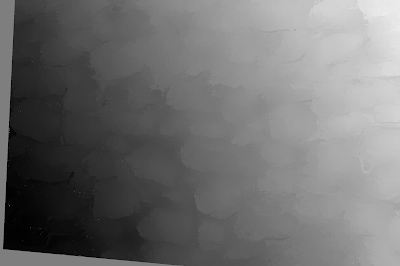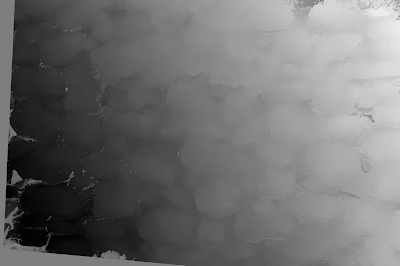## Sunday, December 20, 2015

### 3D Photos - Old stone wall

The stereo pair came from Photosculpt, a company that makes a software which takes a front image and a right image (off by twenty degrees) and produces a 3d texture out of it that can be used in 3d modeling (in programs like Blender).

As always, before even dreaming of getting a depth map, the two images need to be rectified so that the epipolar lines are horizontal, a requirement for most automatic depth map generators. I am gonna use Epipolar Rectification 9 (ER9) to rectify the stereo pair.

This kinda of stereo pairs where there's not much depth and occlusions is perfect for Depth Map Automatic Generator 5b (DMAG5b), a window-based depth map generator that's not edge-aware. If you look closely at the output given by the epipolar rectifier ER9, you can see that it gives a min and max disparities, in this case, -202 and -25. Well, these min and max disparities can be used as input to DMAG5b (and all the other automatic depth map generators) but they have to be reversed, in other words, the min disparity is 25 and the max disparity is 202.Depth map generated by DMAG5b using radius = 17, alpha = 0.9, truncation value (color) = 7.0, truncation value (gradient) = 2.0.

As you can see, there's not a whole lot of occluded pixels (in black).

These could be smoothed out by an edge preserving like Edge Preserving Smoothing 9 (EPS9) or by a Gaussian filter.

Of course, one could have also used our favorite general purpose automatic depth map generator, Depth Map Automatic Generator 7 (DMAG7)Depth map produced by DMAG7 using spatial sample rate = 16, color sample rate = 16, radius = 12, lambda = 0.01.Depth map produced by DMAG7 using spatial sample rate = 16, color sample rate = 16, radius = 12, lambda = 0.1.Depth map produced by DMAG7 using spatial sample rate = 16, color sample rate = 16, radius = 12, lambda = 1.Depth map produced by DMAG7 using spatial sample rate = 32, color sample rate = 16, radius = 12, lambda = 0.1.Depth map produced by DMAG7 using spatial sample rate = 16, color sample rate = 32, radius = 12, lambda = 0.1.

These depth maps (obtained by DMAG7) could probably be smoothed by Edge Preserving Smoothing 7 (EPS7), the so-called recursive domain filter.